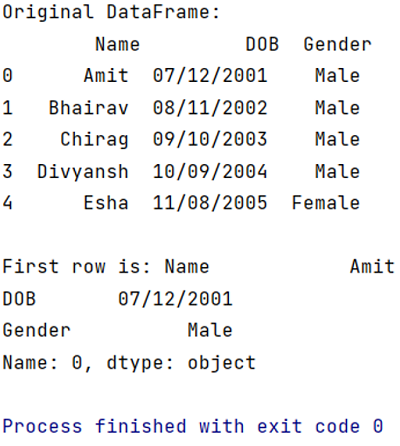# Pandas DataFrame - Get first row value of a given column

Given a DataFrame, we have to get first row value of a given column.
Submitted by Pranit Sharma, on April 27, 2022

Pandas iloc() property allows us to select a row by its index value. Here, the key point is we can also select multiple rows with the help of iloc() property. We can select the specific row using its index value as well as based on a specific column condition.

i in iloc() stands for index. This is also a data selection method but here, we need to pass the proper index as a parameter to select the required row or column. Index is nothing but the integer value ranging from 0 to n-1 which represents the number of rows or columns. We can perform various operations using iloc() property. Inside iloc() property, the index value of the row comes first followed by the number of columns.

Selecting the first row means selecting the index 0. So, we need to pass 0 as an index inside the iloc() property.

To work with Python Pandas, we need to import the pandas library. Below is the syntax,

```import pandas as pd
```

Let us understand with the help of an example.

```# Importing pandas package
import pandas as pd

# Creating a Dictionary
dict = {
'Name':['Amit','Bhairav','Chirag','Divyansh','Esha'],
'DOB':['07/12/2001','08/11/2002','09/10/2003','10/09/2004','11/08/2005'],
'Gender':['Male','Male','Male','Male','Female']
}

# Creating a DataFrame
df = pd.DataFrame(dict)

# Display original DataFrame
print("Original DataFrame:\n",df,"\n")

# Selecting First row
first_row = df.iloc

# Display first row
print("First row is:",first_row)
```

Output:What's New (MCQs)

Top Interview Coding Problems/Challenges!

IncludeHelp's Blogs

Languages: » C » C++ » C++ STL » Java » Data Structure » C#.Net » Android » Kotlin » SQL
Web Technologies: » PHP » Python » JavaScript » CSS » Ajax » Node.js » Web programming/HTML
Solved programs: » C » C++ » DS » Java » C#
Aptitude que. & ans.: » C » C++ » Java » DBMS
Interview que. & ans.: » C » Embedded C » Java » SEO » HR
CS Subjects: » CS Basics » O.S. » Networks » DBMS » Embedded Systems » Cloud Computing
» Machine learning » CS Organizations » Linux » DOS
More: » Articles » Puzzles » News/Updates